Courses

# Centre Of Mass MCQ Level - 2

## 10 Questions MCQ Test Basic Physics for IIT JAM | Centre Of Mass MCQ Level - 2

Description
This mock test of Centre Of Mass MCQ Level - 2 for Physics helps you for every Physics entrance exam. This contains 10 Multiple Choice Questions for Physics Centre Of Mass MCQ Level - 2 (mcq) to study with solutions a complete question bank. The solved questions answers in this Centre Of Mass MCQ Level - 2 quiz give you a good mix of easy questions and tough questions. Physics students definitely take this Centre Of Mass MCQ Level - 2 exercise for a better result in the exam. You can find other Centre Of Mass MCQ Level - 2 extra questions, long questions & short questions for Physics on EduRev as well by searching above.
QUESTION: 1

### A particle of mass m is moving along the x-axis with speed v when it collides with a particle of mass 2m initially at rest. After the collision, the first particle has come to rest and the second particle has split into two equal-mass pieces that are shown in the figure. Which of the following statements correctly describes the speeds of the two pieces? (θ > 0)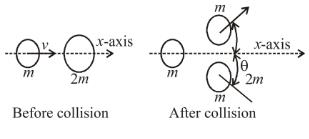Solution:

After collision by momentum conservation :

Along y-axis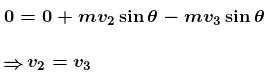Along x-axis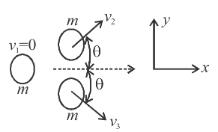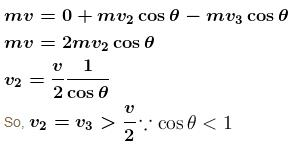The correct answer is: Each piece moves with speed greater than v/2.

QUESTION: 2

### A system of the blocks A and B are connected by an inextensible massless string as shown in the figure. The pulley is massless and frictionless. Initially the system is at rest when, a bullet of mass m moving with a velocity u hits the block B and gets embedded into it. The impulse imparted by tension force to the block of mass 3m is :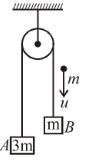Solution:

By conservation of linear momentum along the string.
mu = (m + m + 3m)v
or v = u/5

and impulse on the block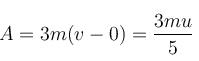The correct answer is: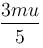QUESTION: 3

### A smooth ball A moving with velocity v collides with another smooth identical ball at rest. After collision both the balls move with same speed with angle between their velocities 60°. No external force acts on the system of balls. The speed of each ball after collision is :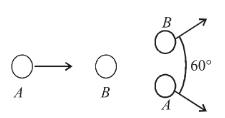Solution:

From conservation of momentum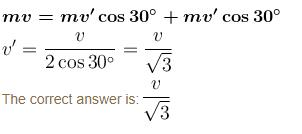QUESTION: 4

A canon shell moving along a straight line burst into two parts. Just after the burst one part moves with momentum 20 Ns making an angle 30° with the original line of motion. The minimum momentum of the other parts of shell just after the burst is :

Solution:

As shown in figure the component of momentum of one shell along initial direction and perpendicular to initial direction are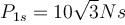and P1y=10 Ns.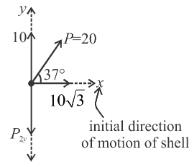For momentum of the system to be zero in y-direction P2y must be 10 Ns. 2nd part of shell may or not have momentum in x-direction.
∴  P2min = 10 Ns.
The correct answer is: 10 Ns

QUESTION: 5

On a smooth carom board, a coin moving in negative y-direction with a speed of 3m/s is being hit at the point (4,6) by a striker moving along negative x-axis. The line joining centres of the coin and the striker just before the collision is parallel to x-axis. After collision the coin goes into the hole located at the origin. Masses of the striker and the coin are equal. Considering the collision to be elastic, the initial and final speeds of the striker in m/s will be: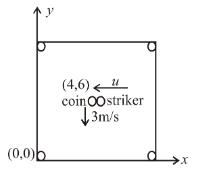Solution:

The line of impact for duration of collision is parallel to x-axis.

The situation of striker and coin just before the collision is given as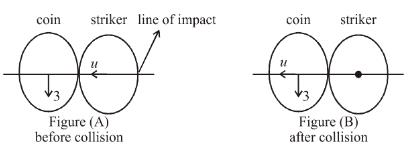Because masses of coin and striker are same, their components of velocities along line of impact shall exchange. Hence the striker comes to rest and the x-y component of velocities of coin are u and 3m/s as shown in figure.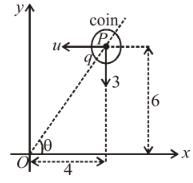For coin to enter hole,

its velocity must be along PO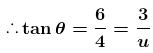or u = 2m/s
The correct answer is: (2, 0)

QUESTION: 6

In given figure, the small block of mass 2m is released from rest when the string is in horizontal position,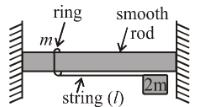Displacement of the ring when string makes an angle θ = 37° with the vertical will be :

Solution:

Taking 'O' as the origin;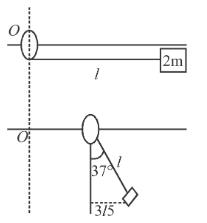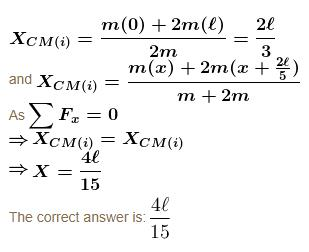QUESTION: 7

A train of mass M is moving on a circular track of radius R with constant speed V. The length of the train is half of the perimeter of the track. The linear momentum of the train will be :

Solution:

If we treat the train as a ring of mass M then its centre of mass will be at a distance

2R/π from the centre of the circle. Velocity of centre of mass is :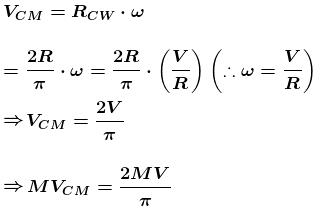As the linear momentum of the system = MVCM

∴ The linear momentum of the train =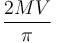The correct answer is:QUESTION: 8

The figure shows a hollow cube of side a of volume V. There is a small chamber of volume V/4 in the cube as shown. This chamber is completely filled by m kg of water. Water leaks through a hole H. The the work done by gravity in this process assuming that the complete water finally lies at the bottom of the cube is :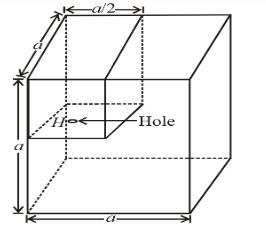Solution:

Let h be the height of water surface, finally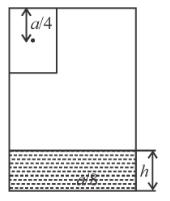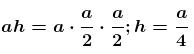∴ C.M. gets lowered by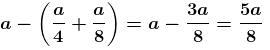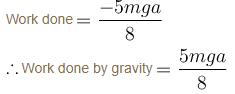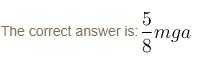QUESTION: 9

AB is and L shaped object fixed on a horizontal smooth table. A ball strikes if at A, gets deflected and restrikes it at B. If the velocity vector before collision is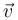and coefficient of restitution of each collision is ‘e’, then the velocity of ball after its second collision at B is :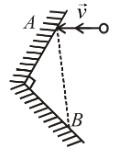Solution: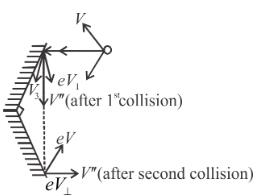During 1st collision perpendicular component of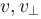becomes e times, while IInd component v|| remains unchanged and similarly for second collision. The end result is that both v|| and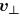becomes e times their initial value and hence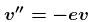(the (–) sign indicates the reversal of direction).

The correct answer is: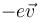QUESTION: 10

A gun is firing 20 balls per seconds of mass 20 gm each on the smooth horizontal table surface ABCD. If the collision is perfectly elastic and balls are striking at the centre of table with a speed 5 m/sec at an angle of 60° with the vertical just before collision, then force exerted by one of the leg on ground is (assume total weight of the table is 0.2 kg and g = 10 m/s2) :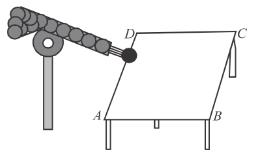Solution:

Force on table due to collision of balls :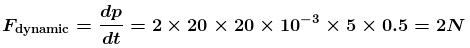Net force one leg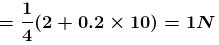The correct answer is: 1 N